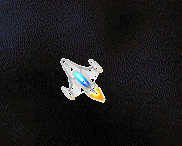## Using small delays in C with SDL ticksRunning a game at 60 frames per second means that handling things like key presses can be interesting. In the game, I call various SDL functions and via a giant switch statement set flags. Then later in the game loop, those flags are used to determine actions

So if you press Q to rotate the player ship anti-clockwise (counter-clockwise to yanks!) without some limiting thing, it would whizz round through 900 degrees each second. (There are 24 rotation angles for the ship, each 15 degrees. 60 x 15 = 900) .

I use a very simple technique to limit it. SDL has a function called SDL_GetTicks that returns the number of ticks since SDL was initialized in the game, i.e. when it started running. A tick is 1/1000th of a second, i.e. a millisecond. By calling this function twice, you can measure a time period. It’s not as precise as the nanosecond CPU clock that I use but for the kind of delays I’m talking about it is more than sufficient.

This is the code that is called each frame in the game loop.

``````void RotatePlayerShip() {
if (rotateFlag && (SDL_GetTicks() - rotTimer > 40)) {
rotTimer = SDL_GetTicks();
if (rotateFlag == 1) // CounterClockwise
{
Player.dir += 23;
Player.dir %= 24;
}
else
if (rotateFlag == 2) // Clockwise
{
Player.dir++;
Player.dir %= 24;
}
}
}``````

Because the game loop syncs to the vertical fly-back, the time between two successive calls of this would be about 16.666 milliseconds. (=1000/60), but the check to see if 40 ticks have passed slows it down to 25 x 15 = 375 degrees rotation per second, i.e. just over one complete revolution which is more manageable than 900/360 = 2.5 full rotations. Plus if you wish rotation speed to be faster just change the 40 to a lower value.

This measured time delay is used quite a few times in the game. You could use it as a means to make the game get harder by having shorter delays on say aliens moving or firing.